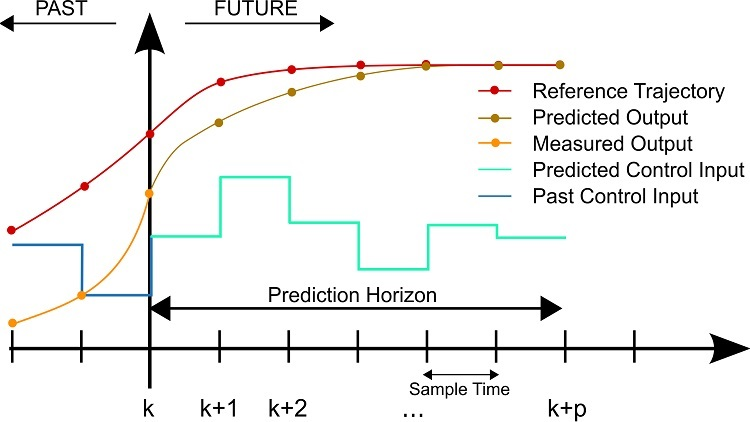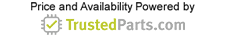Discrete and Process Automation

# Advantages and applications of model predictive control

19 August 2021A discrete MPC scheme. Source: Martin Behrendt/CC BY-SA 3.0

In the 1950s, the predictive control philosophy was developed for process control, which by definition is incredibly sluggish. This control method has been utilized to develop a systemic solution for the oil and chemical industries' multivariable-constrained control problems and is generally known due to its simple design. The advancement of digital signal processors facilitated the introduction of predictive control techniques to electrical engineering applications such as power electronic converters, robotics and others requiring fast variables.

Traditional control methods such as proportional-derivative and proportional-integral-derivative (PID) control may struggle to ensure a variety of complex and nonlinear system characteristics. However, model predictive control (MPC) is one such method that can handle system complexities.

What is MPC?

The majority of control rules, such as PID, don't account for future behavior of control systems. To a certain degree, this may be taken into account by only the predictable dynamics of a closed-loop. However, MPC figures the future behavior directly over a given horizon. As a result, the existing proposed input paths can be limited to those that do not create potential problems in the future.

Consider crossing the street. The information that the lane between you and the other side is devoid of cars is insufficient; you also need to see if there are any vehicles still at a distance that will quickly cross in front of you. So, you are forecasting the likelihood of being struck by a vehicle at some point during your crossing. If there is a high chance of being hit, one keeps standing at the curb. Additionally, while crossing one continues looking, to update forecasts, so that the course around the road can be changed if desired. To forecast the potential behavior of a system, designers must first develop a model of the process's behavior. This model would demonstrate the output's dependency on the current calculated parameter and the current or future inputs.

Most MPC algorithms employ linear models because the forecasts are linear in their reliance on future control decisions, which enables optimization along with the off-line examination of predicted closed-loop behavior. Nonlinear models, on the other hand, could be used in situations where the implicit computational constraint is not a concern and linear approximations are insufficiently precise. MPC only considers the first calculated control input and then recomputes an optimal forecast depending upon the feedback. Therefore, MPC is a model-based, optimal, predictive, iterative and feedback-based control algorithm.

MPC operating principles

MPC is a control algorithm that is built on the concept of moving horizon. Let’s take an example of a demand response power scheduling control process problem. In this case, an optimal power schedule is selected for a given day-ahead optimization plan with a certain optimization horizon T = [0,t] and according to desired parameter predictions such as weather conditions at a starting point t. This starting or initial point t would be less than zero. As a result, the power schedule can be viewed as an optimum trajectory for this particular implementation of demand response power scheduling. But due to the uncertainties inherent in all parameter predictions, the actual power schedule at time tk ≥ 0 can vary from the optimum trajectory.

To catch the above-discussed variation in terms of MPC, another optimization is done at time tk, this time considering the next p potential timeslots, or setting the MPC optimization horizon to T(MPC) = [k, k + p], where p represents the step sizes of MPC (see the diagram above). As a result, the variance is likely to be accounted for by a subsequent optimization of the same goal, so that the actual demand response power plan resumes its optimum trajectory in near future time steps. Nevertheless, since the MPC operation must be reproduced after every p time slot, the cost of applying MPC can be high in terms of computational power. Therefore, researchers have proposed many efficient MPC approaches and research is still going on to further improve the performance of MPC control algorithms.

MPC embodies several characteristics that contribute to its performance as an approach to industrial control design. To begin, it is a simple control algorithm with fundamental concepts that do not require sophisticated mathematics and are "intuitive." Each of the fundamental MPC components can be customized to the specifics of the current problem and it is easy to implement. Its utility is determined by its ability to solve problems such as satisfying control or performance constraints. Finally, it works as demonstrated by several real-world uses in industries, in which MPC is profitable and routinely used.

Applications of MPC

Though MPC applies to virtually every type of problem, it demonstrates its greatest strength while extended to problems such as those involving numerous variables that can be influenced and controlled, a change of control goals or a malfunction of equipment (sensor/actuator), controlled and influenced variables with constraints, and problems with time delays.

Conclusion

MPC is commonly used in the process industry as a control solution with a proven track record. The approach is frequently used in those industrial applications where a high level of uncertainty is involved.

To contact the author of this article, email engineering360editors@globalspec.com